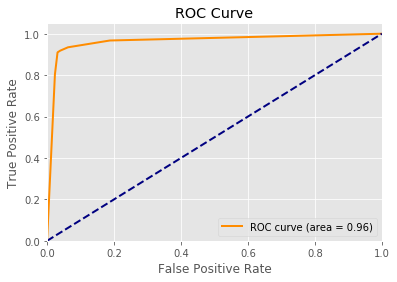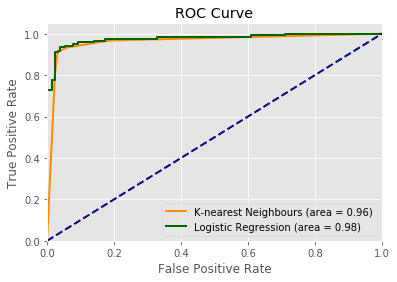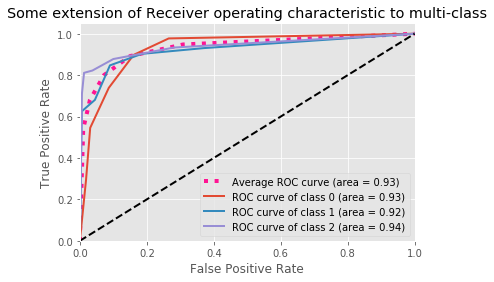# Scoring Classifier Models using scikit-learn

scikit-learn comes with a few methods to help us score our categorical models.

The first is accuracy_score, which provides a simple accuracy score of our model.

In :
from sklearn.metrics import accuracy_score

# True class
y = [0, 0, 1, 1, 0]
# Predicted class
y_hat = [0, 1, 1, 0, 0]

# 60% accuracy
accuracy_score(y, y_hat)

Out:
0.59999999999999998

This works out the same if we have more than just a binary classifier

In :
# True class
y = [0, 1, 2, 1, 0]
# Predicted class
y_hat = [0, 2, 2, 1, 0]

# 80% accuracy
accuracy_score(y, y_hat)

Out:
0.80000000000000004

Of course this doesn’t provide any information about whether the model is has any false positives or false negatives.

We would often use a confusion matrix to find out our type I and type II error rate.

A confusion matrix $C$ is such that $C_{i, j}$ is the number of predictions known to be in group $i$ but predicted to be in group $j$

In :
from sklearn.datasets import make_classification
from sklearn.linear_model import LogisticRegression
from sklearn.model_selection import train_test_split

# Binary Classification
X, y = make_classification(n_samples=1000, n_features=4, n_classes=2)

X_train, X_test, y_train, y_test = train_test_split(X, y, test_size=0.25, random_state=1)

from sklearn.neighbors import KNeighborsClassifier

model = KNeighborsClassifier()
model.fit(X_train, y_train)

y_predict = model.predict(X_test)

In :
from sklearn.metrics import confusion_matrix

confusion_matrix(y_test, y_predict)

Out:
array([[123,   5],
[ 10, 112]])

Because the confusion matrix is such A $C_{i, j}$ is the number of predictions known to be in group $i$ but predicted to be in group $j$, we have:

• $C_{0, 0} = 123$ – True Negatives, response 0, predicted 0
• $C_{1, 0} = 10$ – False Negatives, response 1, predicted 0
• $C_{0, 1} = 5$ – False Positives, response 0, predicted 1
• $C_{1, 1} = 112$ – True Positives, response 1, predicted 1

We can plot this using an ROC curve, where we plot the True Positive rate against the False Positive rate, in which a large area under the curve is more favourable.

In :
from sklearn.metrics import roc_curve, auc
import matplotlib.pyplot as plt
plt.style.use('ggplot')
%matplotlib inline

y_predict_probabilities = model.predict_proba(X_test)[:,1]

fpr, tpr, _ = roc_curve(y_test, y_predict_probabilities)
roc_auc = auc(fpr, tpr)

plt.figure()
plt.plot(fpr, tpr, color='darkorange',
lw=2, label='ROC curve (area = %0.2f)' % roc_auc)
plt.plot([0, 1], [0, 1], color='navy', lw=2, linestyle='--')
plt.xlim([0.0, 1.0])
plt.ylim([0.0, 1.05])
plt.xlabel('False Positive Rate')
plt.ylabel('True Positive Rate')
plt.title('ROC Curve')
plt.legend(loc="lower right")
plt.show()This curve hugs the top left and has a very high area under the curve (AUC) so this model has performed well on our test data set.

The dashed blue line approximates the line if a random guess (coin-flip) were taken.

### Comparing Classifiers

We can compare the performance of a classifier against each other to see which performs better using ROC curves

In :
from sklearn.linear_model import LogisticRegression

lr_model = LogisticRegression()
lr_model.fit(X_train, y_train)

lr_predict_probabilities = lr_model.predict_proba(X_test)[:,1]

lr_fpr, lr_tpr, _ = roc_curve(y_test, lr_predict_probabilities)
lr_roc_auc = auc(lr_fpr, lr_tpr)

plt.figure()
plt.plot(fpr, tpr, color='darkorange',
lw=2, label='K-nearest Neighbours (area = %0.2f)' % roc_auc)
plt.plot(lr_fpr, lr_tpr, color='darkgreen',
lw=2, label='Logistic Regression (area = %0.2f)' % lr_roc_auc)
plt.plot([0, 1], [0, 1], color='navy', lw=2, linestyle='--')
plt.xlim([0.0, 1.0])
plt.ylim([0.0, 1.05])
plt.xlabel('False Positive Rate')
plt.ylabel('True Positive Rate')
plt.title('ROC Curve')
plt.legend(loc="lower right")
plt.show()So the 2 models perform similarly well on this occasion.

### Multiple Classes

So we’ve seen a binary classifier, but what if we had multiple classes (3 or more). The same applies here. We can do a confusion matrix in which we see how well our model has performed

In :
from sklearn.multiclass import OneVsRestClassifier
# 3-class Classification
X, y = make_classification(1000, n_features=2, n_redundant=0, n_informative=2,
n_clusters_per_class=1, n_classes=3)

X_train, X_test, y_train, y_test = train_test_split(X, y, test_size=0.25, random_state=1)

model = KNeighborsClassifier()
model.fit(X_train, y_train)

y_predict = model.predict(X_test)

confusion_matrix(y_test, y_predict)

Out:
array([[72, 11,  5],
[10, 61,  1],
[ 7,  9, 74]])

Because the confusion matrix is such A $C_{i, j}$ is the number of predictions known to be in group $i$ but predicted to be in group $j$

Our True positives are on the diagonal axis and are the largest numbers here.

The False Negatives are the sum of the other values along the rows.

The False Positives are the sum of the other values down the columns.

To plot an ROC curve here, we actually have to plot multiple ROC curves and we’ll take an average of

In :
import numpy as np

y_predict_proba = model.predict_proba(X_test)

# Compute ROC curve and ROC AUC for each class
n_classes = 3
fpr = dict()
tpr = dict()
roc_auc = dict()
all_y_test_i = np.array([])
all_y_predict_proba = np.array([])
for i in range(n_classes):
y_test_i = map(lambda x: 1 if x == i else 0, y_test)
all_y_test_i = np.concatenate([all_y_test_i, y_test_i])
all_y_predict_proba = np.concatenate([all_y_predict_proba, y_predict_proba[:, i]])
fpr[i], tpr[i], _ = roc_curve(y_test_i, y_predict_proba[:, i])
roc_auc[i] = auc(fpr[i], tpr[i])

# Compute micro-average ROC curve and ROC area
fpr["average"], tpr["average"], _ = roc_curve(all_y_test_i, all_y_predict_proba)
roc_auc["average"] = auc(fpr["average"], tpr["average"])

# Plot average ROC Curve
plt.figure()
plt.plot(fpr["average"], tpr["average"],
label='Average ROC curve (area = {0:0.2f})'
''.format(roc_auc["average"]),
color='deeppink', linestyle=':', linewidth=4)

# Plot each individual ROC curve
for i in range(n_classes):
plt.plot(fpr[i], tpr[i], lw=2,
label='ROC curve of class {0} (area = {1:0.2f})'
''.format(i, roc_auc[i]))

plt.plot([0, 1], [0, 1], 'k--', lw=2)
plt.xlim([0.0, 1.0])
plt.ylim([0.0, 1.05])
plt.xlabel('False Positive Rate')
plt.ylabel('True Positive Rate')
plt.title('Some extension of Receiver operating characteristic to multi-class')
plt.legend(loc="lower right")
plt.show()